# Vlookup to Find Last Matching Value

Lookup Function to Get Last Matching Value in Microsoft Excel

If you are wondering what formula should be used in order to get the last matching value in Excel to return the corresponding cell value, then this article is for you.

In this article, we will learn to use lookup function to get last matching value out of multiple values.

Question): I need a formula to look up the conditional value in a specific column & find the last occurred item & then return the corresponding value.

The data we will consider in this example has some names in column A, couple of them are repeated to have better understanding of this example; the second column has sale quantity.

Following is the snapshot of the sample data:

• The formula should first of all check should check the number of times the criteria text found & then provide the adjacent value.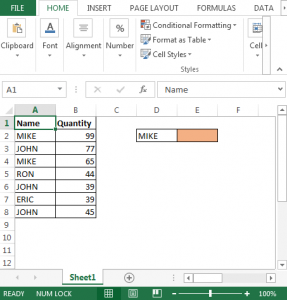• In cell E2, the formula is
• =LOOKUP(2,1/(\$A\$2:\$A\$8=D2),\$B\$2:\$B\$8)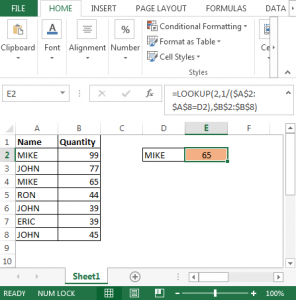Code Explanation:

• This part (\$A\$2:\$A\$8=D2) evaluates to an array of TRUEs/FALSEs.
• 1 should be divided each of these evaluates to an array of 1's and #DIV/0! error (because 1/True is like 1/1, which equals 1 and 1/False is like 1/0 which results in an error.
• The Lookup() function looks for the last entry that is less than or equal to the Lookup value... so looking for a 2 in an array of 1's and #DIV/0!'s will result in returning the last 1 in the array
• This is then matched to the Result Vector; B2:B8 to pull the coinciding value.

To test the above formula, we can either change the conditional text or change in source data.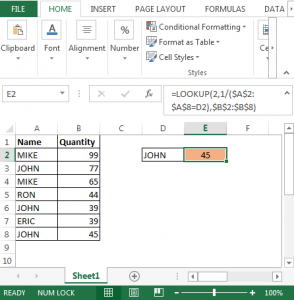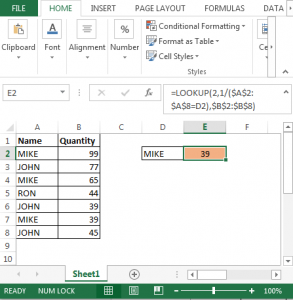In this way, find the quantity sale by last occurrence of the searched name.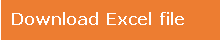Related Articles:

Use INDEX and MATCH to Lookup Value in Excel

How to use the LOOKUP function in Excel

Lookup Value with Multiple Criteria in Excel

Popular Articles

50 Excel Shortcut to Increase Your Productivity: Get faster at your task. These 50 shortcuts will make you work even faster on Excel.

How to use the VLOOKUP Function in Excel: This is one of the most used and popular functions of excel that is used to lookup value from different ranges and sheets.

How to use the COUNTIF function in Excel: Count values with conditions using this amazing function. You don't need to filter your data to count specific values. Countif function is essential to prepare your dashboard.

How to use the SUMIF Function in ExcelThis is another dashboard essential function. This helps you sum up values on specific conditions.

1.2.hi.
can you help to solve the problem,
I am selling the same product repeatedly. I have two columns that are Total Quantity and Available Quantity. in each time how can I know the presently available quantity.

3.It seems to return #N/A if there is only one occurrence of the lookup value.
How can you make this work when some lookup values have multiple occurrences and some only occur once?

4.Thanks, it was a great help that I didn't have to invent it for my own 🙂

5.I am getting #NA when tried with above example. When I tried to evaluate formula...it worked till below step
1 should be divided each of these evaluates to an array of 1?s and #DIV/0! error (because 1/True is like 1/1, which equals 1 and 1/False is like 1/0 which results in an error.

6.Christofer Wimberly

I am trying to pull the value from the next sheet over. The cell which i am trying to pull the value from is calculated via a formula.
When the calculated cell is filled the formula =LOOKUP(2,1/('CRM'!\$O\$5:\$O\$100=Inventory!B12),'CRM'!\$AE14:\$AE\$1000) returns a blank despite the cell having a numerical value

•Christofer Wimberly

Never mind I figured it out. Thank you!

•can you explain how you resolved it. also can you explain your formula i too have a similar issue

•This formula works on the one property of LOOKUP function. If the lookup function does not finds the lookup value in given range, it returns the last value that is less than the lookup value.

7.This is brilliant stuff! Very smart of you!

8.Thank you! Just what I needed!

9.Excellent, Excel is Passion.........Great work.

Terms and Conditions of use

The applications/code on this site are distributed as is and without warranties or liability. In no event shall the owner of the copyrights, or the authors of the applications/code be liable for any loss of profit, any problems or any damage resulting from the use or evaluation of the applications/code.